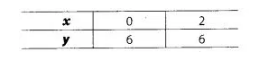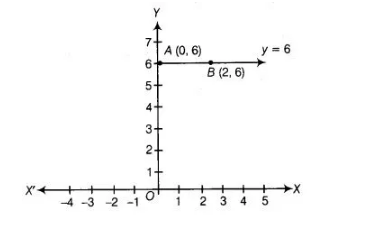# The graph of y = 6 is a Line`
Question:

The graph of y = 6 is a Line

(a) parallel to X-axis at a distance 6 units from the origin

(b) parallel to Y-axis at a distance 6 units from the origin

(c) making an intercept 6 on the X-axis

(d) making an intercept 6 on both axes

Solution:

(a) Given equation of line can be written as, a . x + 1 . y = 6

To draw the graph of above equation, we need atleast two solutions. When x = 0, then y = 6 When x =2, then y = 6Here, we find two points A (0, 6) and B (2, 6). So, draw the graph, by plotting the points and joining the line AB.